# New sharp bounds for logarithmic mean and identric mean

## Abstract

For $x,y>0$ with $x\ne y$, let $L=L\left(x,y\right)$, $I=I\left(x,y\right)$, $A=A\left(x,y\right)$, $G=G\left(x,y\right)$, ${A}_{r}={A}^{1/r}\left({x}^{r},{y}^{r}\right)$ denote the logarithmic mean, identric mean, arithmetic mean, geometric mean and r-order power mean, respectively. We find the best constant $p,q>0$ such that the inequalities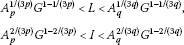hold, respectively. From them some new inequalities for means are derived. Lastly, our new lower bound for the logarithmic mean is compared with several known ones, which shows that our results are superior to others.

MSC: 26D07, 26E60, 05A15, 15A18.

## 1 Introduction

The logarithmic and identric means of two positive real numbers x and y with $x\ne y$ are defined by

$L=L\left(x,y\right)=\frac{x-y}{lnx-lny}\phantom{\rule{1em}{0ex}}\text{and}\phantom{\rule{1em}{0ex}}I=I\left(x,y\right)={e}^{-1}{\left(\frac{{x}^{x}}{{y}^{y}}\right)}^{1/\left(x-y\right)},$

respectively. The power mean of order r of the positive real numbers x and y is defined by

The main properties of these means are given in . In particular, the function $r↦{A}_{r}\left(x,y\right)$ ($x\ne y$) is continuous and strictly increasing on . As special cases, the arithmetic mean and geometric mean are $A=A\left(x,y\right)={A}_{1}\left(x,y\right)$ and $G=G\left(x,y\right)={A}_{0}\left(x,y\right)$, respectively.

In the recent past, the logarithmic mean and the identric mean have been the subject of intensive research. Ostle and Terwilliger  and Karamata  first proved that

$G
(1.1)

This result, or a part of it, has been rediscovered and reproved many times (see, e.g., ).

In 1974 Lin  obtained an important refinement of the above inequalities:

$G
(1.2)

and proved that the number $1/3$ cannot be replaced by a smaller one. A sharpness of the second inequality in (1.2) has been shown by Neuman . The following inequality is due to Carlson :

$L>\sqrt{{A}_{1/2}G}.$
(1.3)

In , the authors present a very nice double inequality, that is,

${A}^{1/3}{G}^{2/3}
(1.4)

Using a new method, Wang and Wang  proved that

$L>{A}_{p}^{1-p}{G}^{p}$
(1.5)

holds for $p=0,1/2,1/3$. Chen and Wang  pointed out this inequality is true for all real numbers p. Only when $p\in \left(0,1\right)$, however, the inequality ${A}_{p}^{1-p}{G}^{p}>G$ would be true. In 2009, another better lower bound for L was given by Zhu , that is,

$L>{\left(\frac{7}{15}A+\frac{8}{15}G\right)}^{5/7}{G}^{2/7}>{A}_{1/2}^{2/3}{G}^{1/3}>{\left(\frac{2}{3}A+\frac{1}{3}G\right)}^{1/2}{G}^{1/2}>{A}^{1/3}{G}^{2/3}.$
(1.6)

The following lower bound for L in terms of I and G is due to Alzer :

$L>\sqrt{IG}.$
(1.7)

Very recently, Yang  showed that

$L>{A}_{1/2}^{2/3}{G}^{1/3}>\sqrt{IG}>{A}^{1/3}{G}^{2/3}.$
(1.8)

For the identric mean I, Stolarsky [17, 18] and Pittenger  presented lower and upper bounds for I as follows: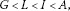(1.9)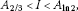(1.10)

and the constants $2/3$ and ln2 are the best possible. Inequalities (1.9) were also rediscovered by Yang . The following result is due to Sándor :

$I>\frac{2A+G}{3}>{A}^{2/3}{G}^{1/3}.$
(1.11)

Other inequalities for L and I and their applications can be found in the literature .

The aim of this paper is to find the best $p,q\in \mathbb{R}$ such that the inequalities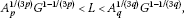(1.12)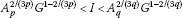(1.13)

hold.

It is easy to check that both the functions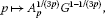(1.14)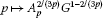(1.15)

are even on $\left(-\mathrm{\infty },\mathrm{\infty }\right)$, and therefore we assume that $p,q>0$ in what follows.

Our main results are stated as follows.

Theorem 1 Let $p,q>0$. Then inequalities (1.12) hold for all $x,y>0$ with $x\ne y$ if and only if $p\ge {p}_{0}=1/\sqrt{5}$ and $0, and the function $p↦{A}_{p}^{1/\left(3p\right)}{G}^{1-1/\left(3p\right)}$ is decreasing on $\left(0,\mathrm{\infty }\right)$.

Theorem 2 Let $p,q>0$. Then inequalities (1.13) hold for all $x,y>0$ with $x\ne y$ if and only if $p\ge 2/3$ and $0, and the function $p↦{A}_{p}^{2/\left(3p\right)}{G}^{1-2/\left(3p\right)}$ is decreasing on $\left(0,\mathrm{\infty }\right)$.

We will prove two theorems above by hyperbolic function theory. For this end, we need the following lemma, which tells us an inequality for bivariate homogeneous means can be equivalently changed into the form of hyperbolic functions.

Lemma 1 Let $M\left(x,y\right)$ be a homogeneous mean of positive arguments x and y. Then

$M\left(x,y\right)=\sqrt{xy}M\left({e}^{t},{e}^{-t}\right),$

where $t=\frac{1}{2}ln\left(x/y\right)$.

By symmetry, we assume that $x>y>0$. Then we have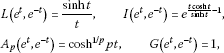where $t=\frac{1}{2}ln\left(x/y\right)>0$. And then, due to Lemma 1, Theorem 1 and Theorem 2 can be restated as equivalent ones, respectively.

Theorem 1′ Let $p,t>0$. Then the inequality

$\frac{sinht}{t}>{\left(coshpt\right)}^{1/\left(3{p}^{2}\right)}$
(1.16)

holds for all $t>0$ if and only if $p\ge {p}_{0}=1/\sqrt{5}$ and the function $p↦{\left(coshpt\right)}^{1/\left(3{p}^{2}\right)}$ is decreasing on $\left(0,\mathrm{\infty }\right)$. Inequality (1.16) is reversed if and only if $0.

Theorem 2′ Let $p,t>0$. Then the inequality

${e}^{\frac{tcosht}{sinht}-1}>{\left(coshpt\right)}^{2/\left(3{p}^{2}\right)}$
(1.17)

holds for all $t>0$ if and only if $p\ge 2/3$ and the function $p↦{\left(coshpt\right)}^{2/\left(3{p}^{2}\right)}$ is decreasing on $\left(0,\mathrm{\infty }\right)$. Inequality (1.17) is reversed if and only if $0.

Therefore, we will prove Theorem 1′ and Theorem 2′ instead of Theorem 1 and Theorem 2 in the sequel.

## 2 Proof of Theorem 1′

In order to prove Theorem 1′, we first give the following lemmas.

Lemma 2 For $t>0$, let the function $U:\left(0,\mathrm{\infty }\right)↦\left(0,\mathrm{\infty }\right)$ be defined by

$U\left(p\right)={p}^{-2}lncoshpt.$
(2.1)

Then U is decreasing on $\left(0,\mathrm{\infty }\right)$ with

$\underset{p\to {0}^{+}}{lim}U\left(p\right)=\frac{1}{2}{t}^{2},\phantom{\rule{2em}{0ex}}\underset{p\to \mathrm{\infty }}{lim}U\left(p\right)=0.$

Proof

Differentiation yields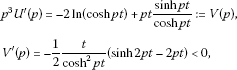which implies that V is decreasing on $\left(0,\mathrm{\infty }\right)$, and so $V\left(p\right)<{lim}_{p\to 0}V\left(p\right)=0$. Therefore, ${U}^{\mathrm{\prime }}\left(p\right)<0$, that is to say, U is decreasing on $\left(0,\mathrm{\infty }\right)$. And, by L’Hospital’s rule, we have

$\underset{p\to 0}{lim}U\left(p\right)=\underset{p\to 0}{lim}\frac{1}{2p}\frac{tsinpt}{\left(coshpt\right)}=\frac{1}{2}{t}^{2},\phantom{\rule{2em}{0ex}}\underset{p\to \mathrm{\infty }}{lim}U\left(p\right)=\underset{p\to \mathrm{\infty }}{lim}\frac{1}{2p}\frac{tsinpt}{\left(coshpt\right)}=0,$

which proves the lemma. □

Remark 1 From Lemmas 1 and 2 it follows that the function $p↦{\left({A}_{p}/G\right)}^{1/p}$ is decreasing on $\left(0,\mathrm{\infty }\right)$, and

$\underset{p\to 0}{lim}{\left({A}_{p}/G\right)}^{1/p}=Gexp\left(\frac{1}{8}{ln}^{2}\left(x/y\right)\right),\phantom{\rule{2em}{0ex}}\underset{p\to \mathrm{\infty }}{lim}{\left({A}_{p}/G\right)}^{1/p}=1.$

Since

${A}_{p}^{1/\left(3p\right)}{G}^{1-1/\left(3p\right)}={\left({\left({A}_{p}/G\right)}^{1/p}\right)}^{1/3}G,\phantom{\rule{2em}{0ex}}{A}_{p}^{2/\left(3p\right)}{G}^{1-2/\left(3p\right)}={\left({\left({A}_{p}/G\right)}^{1/p}\right)}^{2/3}G,$

so are the functions defined by (1.14) and (1.15), and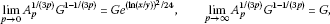(2.2)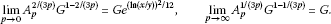(2.3)

Lemma 3 Let $p>0$ and let $f:\left(0,\mathrm{\infty }\right)↦\left(-\mathrm{\infty },\mathrm{\infty }\right)$ be the function defined by

$f\left(t\right)=ln\frac{sinht}{t}-\frac{1}{3{p}^{2}}lncoshpt.$
(2.4)

Then we have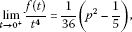(2.5)(2.6)

Proof Using L’Hospital’s rule gives (2.5). To obtain (2.6), we write $f\left(t\right)$ as

$f\left(t\right)=t\left(1-\frac{1}{3p}-\frac{lnt}{t}\right)+ln\frac{1-{e}^{-2t}}{2}-\frac{1}{3{p}^{2}}ln\frac{1+{e}^{-2pt}}{2},$

from which (2.6) easily follows.

This lemma is proved. □

Lemma 4 For $p>0$, let f be defined by (2.4). Then f is increasing if $p\ge 1/\sqrt{5}$ and decreasing if $0.

Proof

Differentiation yields

$\begin{array}{rcl}{f}^{\mathrm{\prime }}\left(t\right)& =& \frac{t}{sinht}\left(\frac{1}{t}cosht-\frac{1}{{t}^{2}}sinht\right)-\frac{1}{3pcoshpt}sinhpt\\ =& \frac{-3pcoshptsinht-tsinhptsinht+3ptcoshptcosht}{3ptcoshptsinht}\\ :=& \frac{{f}_{1}\left(t\right)}{3ptcoshptsinht},\end{array}$
(2.7)

where

${f}_{1}\left(t\right)=-3pcoshptsinht-tsinhptsinht+3ptcoshptcosht.$

Using ‘product into sum’ formula for hyperbolic functions gives

$\begin{array}{rcl}{f}_{1}\left(t\right)& =& \left(\frac{1}{2}+\frac{3}{2}p\right)tcosht\left(p-1\right)+\frac{3}{2}psinht\left(p-1\right)\\ +\left(\frac{3}{2}p-\frac{1}{2}\right)tcosht\left(p+1\right)-\frac{3}{2}psinht\left(p+1\right),\end{array}$

and expanding ${f}_{1}\left(t\right)$ in power series gives

$\begin{array}{rcl}{f}_{1}\left(t\right)& =& \left(\frac{1}{2}+\frac{3}{2}p\right)\sum _{1}^{\mathrm{\infty }}\frac{{\left(p-1\right)}^{2n-2}{t}^{2n-1}}{\left(2n-2\right)!}+\frac{3}{2}p\sum _{1}^{\mathrm{\infty }}\frac{{\left(p-1\right)}^{2n-1}{t}^{2n-1}}{\left(2n-1\right)!}\\ +\left(\frac{3}{2}p-\frac{1}{2}\right)\sum _{1}^{\mathrm{\infty }}\frac{{\left(p+1\right)}^{2n-2}{t}^{2n-1}}{\left(2n-2\right)!}-\frac{3}{2}p\sum _{1}^{\mathrm{\infty }}\frac{{t}^{2n-1}{\left(p+1\right)}^{2n-1}}{\left(2n-1\right)!}\\ :=& \sum _{1}^{\mathrm{\infty }}\frac{u\left(p,n\right){a}_{n}}{2\left(2n-1\right)!}{\left(p+1\right)}^{2n-2}{t}^{2n-1},\end{array}$
(2.8)

where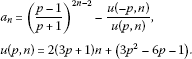It is easy to check that

$u\left(p,n\right)=\left(6p+2\right)\left(n-1+\frac{3{p}^{2}+1}{6p+2}\right)>0$
(2.9)

and

${a}_{n+1}={\left(\frac{p-1}{p+1}\right)}^{2}{a}_{n}+v\left(n,p\right),$
(2.10)

where

$\begin{array}{rcl}v\left(n,p\right)& =& {\left(\frac{p-1}{p+1}\right)}^{2}\frac{u\left(-p,n\right)}{u\left(p,n\right)}-\frac{u\left(-p,n+1\right)}{u\left(p,n+1\right)}\\ :=& \frac{16p\left(n-1\right)}{{\left(p+1\right)}^{2}u\left(p,n\right)u\left(p,n+1\right)}w\left(n,p\right),\end{array}$
(2.11)

here

$w\left(n,p\right)=3\left(3n-1\right){p}^{2}-\left(n+1\right).$
(2.12)

Now we are ready to prove desired results.

(i) We first prove that f is increasing if $p\ge 1/\sqrt{5}$. To this end, by (2.7) in combination with (2.8) and (2.9), it suffices to show that ${a}_{n}\ge 0$ for $n\in \mathbb{N}$. We easily check that ${a}_{1}={a}_{2}=0$ and

${a}_{3}=\frac{16p\left(5{p}^{2}-1\right)}{{\left(p+1\right)}^{4}\left(3{p}^{2}+12p+5\right)}\ge 0.$
(2.13)

Assume that ${a}_{n}>0$ for $n\ge 3$. From (2.12) it is easy to see that

$w\left(n,p\right)\ge w\left(n,\frac{1}{\sqrt{5}}\right)=\frac{4}{5}\left(n-2\right)>0,$

which together with (2.11) yields $v\left(n,p\right)>0$, and from (2.10) it is derived that ${a}_{n+1}>0$. By mathematical induction, we conclude that ${a}_{n}\ge 0$ for $n\in \mathbb{N}$, which proves part one of this lemma.

(ii) Next we show that f is decreasing if $p\le 1/3$. Likewise, it needs to be shown that ${a}_{n}\le 0$ for $n\in \mathbb{N}$. As mentioned previously, ${a}_{1}={a}_{2}=0$ but

${a}_{3}=\frac{16p\left(5{p}^{2}-1\right)}{{\left(p+1\right)}^{4}\left(3{p}^{2}+12p+5\right)}<0.$

Suppose that ${a}_{n}<0$ for $n\ge 3$. We have

$w\left(n,p\right)\le w\left(n,\frac{1}{3}\right)=-\frac{4}{3}<0,$

which leads to $v\left(n,p\right)<0$, and from (2.10) we have ${a}_{n+1}<0$ for $n\ge 3$. By mathematical induction, it is obtained that ${a}_{n}\le 0$ for $n\in \mathbb{N}$.

This completes the proof. □

Now we prove Theorem 1′.

Proof of Theorem 1′ It is clear that (1.16) (or its reverse inequality) is equivalent to $f\left(t\right)>0$ (or <0), where $f\left(t\right)$ is defined by (2.4).

(i) We first show that $f\left(t\right)>0$ for all $t>0$ if and only if $p\ge 1/\sqrt{5}$. If $f\left(t\right)>0$ for all $t>0$, then by (2.5) and (2.6) we have

$\left\{\begin{array}{c}{lim}_{t\to {0}^{+}}\frac{f\left(t\right)}{{t}^{4}}=\frac{1}{36}\left({p}^{2}-\frac{1}{5}\right)\ge 0,\hfill \\ {lim}_{t\to \mathrm{\infty }}\frac{f\left(t\right)}{t}=\frac{1}{p}\left(p-\frac{1}{3}\right)\ge 0,\hfill \end{array}$

which yields $p\ge 1/\sqrt{5}$.

Conversely, if $p\ge 1/\sqrt{5}$, then by Lemma 4 f is increasing on $\left(0,\mathrm{\infty }\right)$, hence

$f\left(t\right)>\underset{t\to {0}^{+}}{lim}f\left(t\right)=0$

for all $t>0$.

(ii) Next we prove that $f\left(t\right)<0$ for all $t>0$ if and only if $p\le 1/3$. If $f\left(t\right)<0$ for all $t>0$, then by (2.5) and (2.6) we have

$\left\{\begin{array}{c}{lim}_{t\to {0}^{+}}\frac{f\left(t\right)}{{t}^{4}}=\frac{1}{36}\left({p}^{2}-\frac{1}{5}\right)\le 0,\hfill \\ {lim}_{t\to \mathrm{\infty }}\frac{f\left(t\right)}{t}=\frac{1}{p}\left(p-\frac{1}{3}\right)\le 0,\hfill \end{array}$

which leads to $0.

Conversely, if $0, then from the monotonicity of f by Lemma 4 we conclude that

$f\left(t\right)<\underset{t\to {0}^{+}}{lim}f\left(t\right)=0$

for all $t>0$.

(iii) Lastly, from Lemma 2 we easily conclude that the function $p↦{\left(coshpt\right)}^{1/\left(3{p}^{2}\right)}$ is decreasing on $\left(0,\mathrm{\infty }\right)$.

Thus the proof is accomplished. □

## 3 Proof of Theorem 2′

The following lemmas are useful.

Lemma 5 Let $p>0$ and let $g:\left(0,\mathrm{\infty }\right)↦\left(-\mathrm{\infty },\mathrm{\infty }\right)$ be the function defined by

$g\left(t\right)=\left(\frac{tcosht}{sinht}-1\right)-\frac{2}{3{p}^{2}}ln\left(coshpt\right).$
(3.1)

Then we have(3.2)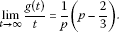(3.3)

Proof Since $g\left(t\right)\to 0$ as $t\to {0}^{+}$, using L’Hospital’s rule yields (3.2). To obtain (3.3), we have to change $g\left(t\right)$ as follows:

$g\left(t\right)=\left(1-\frac{2}{3p}\right)t+\left(\frac{2t}{{e}^{2t}-1}-1\right)-\frac{2}{3{p}^{2}}ln\frac{1+{e}^{-2pt}}{2},$
(3.4)

from which (3.3) follows.

Thus the lemma is proved. □

Lemma 6 For $p>0$, let the function g be defined by (3.1). Then g is increasing if $p\ge 2/3$ and decreasing if $0.

Proof

Differentiation yields

${g}^{\mathrm{\prime }}\left(t\right)=-\frac{{g}_{1}\left(t\right)}{3pcoshpt{sinh}^{2}t},$
(3.5)

where

$\begin{array}{rcl}{g}_{1}\left(t\right)& =& 2sinhpt{sinh}^{2}t-3pcoshptcoshtsinht\\ +3ptcoshpt{cosh}^{2}t-3ptcoshpt{sinh}^{2}t.\end{array}$

Using ‘product into sum’ formula for hyperbolic functions and expanding in power series give

$\begin{array}{rcl}{g}_{1}\left(t\right)& =& \left(\frac{1}{2}+\frac{3}{4}p\right)sinht\left(p-2\right)+\left(\frac{1}{2}-\frac{3}{4}p\right)sinht\left(p+2\right)-sinhpt+3ptcoshpt\\ =& \left(\frac{1}{2}+\frac{3}{4}p\right)\sum _{1}^{\mathrm{\infty }}\frac{{t}^{2n-1}{\left(p-2\right)}^{2n-1}}{\left(2n-1\right)!}-\left(\frac{3}{4}p-\frac{1}{2}\right)\sum _{1}^{\mathrm{\infty }}\frac{{t}^{2n-1}{\left(p+2\right)}^{2n-1}}{\left(2n-1\right)!}\\ -\sum _{1}^{\mathrm{\infty }}\frac{{t}^{2n-1}{p}^{2n-1}}{\left(2n-1\right)!}+3p\sum _{1}^{\mathrm{\infty }}\frac{{t}^{2n-1}{p}^{2n-2}}{\left(2n-2\right)!}\\ :=& \sum _{1}^{\mathrm{\infty }}\frac{{b}_{n}}{\left(2n-1\right)!}{p}^{2n-1}{t}^{2n-1},\end{array}$
(3.6)

where

${b}_{n}=\left(\frac{1}{2}+\frac{3}{4}p\right){\left(1-\frac{2}{p}\right)}^{2n-1}-\left(\frac{3}{4}p-\frac{1}{2}\right){\left(1+\frac{2}{p}\right)}^{2n-1}+2\left(3n-2\right).$
(3.7)

We find that

${b}_{n+1}={\left(1-\frac{2}{p}\right)}^{2}{b}_{n}+h\left(n,p\right),$
(3.8)

where

$h\left(n,p\right)=-\frac{2\left(3p-2\right)}{p}{\left(1+\frac{2}{p}\right)}^{2n-1}+\frac{24\left(p-1\right)n+2\left(3{p}^{2}-8p+8\right)}{{p}^{2}}.$

We claim that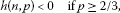(3.9)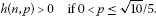(3.10)

Indeed, applying the binomial expansion gives

${\left(1+\frac{2}{p}\right)}^{2n-1}\ge 1+\left(2n-1\right)\frac{2}{p}+\frac{\left(2n-1\right)\left(2n-2\right)}{2!}{\left(\frac{2}{p}\right)}^{2}.$

Hence, if $p\ge 2/3$, then we get

$\begin{array}{rcl}h\left(n,p\right)& <& -\frac{2\left(3p-2\right)}{p}\left(1+\left(2n-1\right)\frac{2}{p}+\frac{\left(2n-1\right)\left(2n-2\right)}{2!}{\left(\frac{2}{p}\right)}^{2}\right)\\ +\frac{24\left(p-1\right)n+2\left(3{p}^{2}-8p+8\right)}{{p}^{2}}\\ =& \frac{16}{{p}^{3}}\left(n-1\right)\left(\left(3n-1\right)\left(\frac{2}{3}-p\right)-\frac{1}{3}\right)<0,\end{array}$

that is, (3.9) holds. If $0, then

$\begin{array}{rcl}h\left(n,p\right)& >& -\frac{2\left(3p-2\right)}{p}\left(1+\left(2n-1\right)\frac{2}{p}+\frac{\left(2n-1\right)\left(2n-2\right)}{2!}{\left(\frac{2}{p}\right)}^{2}\right)\\ +\frac{24\left(p-1\right)n+2\left(3{p}^{2}-8p+8\right)}{{p}^{2}}\\ =& \frac{16}{{p}^{3}}\left(n-1\right)\left(\left(3n-1\right)\left(\frac{2}{3}-p\right)-\frac{1}{3}\right)\\ \ge & 16{\left(\frac{5}{\sqrt{10}}\right)}^{3}\left(n-1\right)\left(\left(3n-1\right)\left(\frac{2}{3}-\frac{\sqrt{10}}{5}\right)-\frac{1}{3}\right)\\ =& 40\left(\sqrt{10}-3\right)\left(n-1\right)\left(n-\frac{4+\sqrt{10}}{2}\right)>0\end{array}$

for $n\ge 4$, which in combination with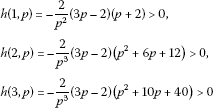Now we are in a position to prove our results.

(i) We first prove that g is increasing if $p\ge 2/3$. For this end, it is enough to show that ${b}_{n}\le 0$ by (3.5) and (3.6). Indeed, we have ${b}_{1}={b}_{2}=0$ and ${b}_{3}=-16{p}^{-4}\left(5{p}^{2}-2\right)<0$. Suppose that ${b}_{n}\le 0$ for $n\ge 4$. From (3.8) and (3.9) we have ${b}_{n+1}<0$, which proves part one of this lemma by mathematical induction.

(ii) Next we prove that g is decreasing if $0. It suffices to prove that ${b}_{n}\ge 0$. We have seen that ${b}_{1}={b}_{2}=0$, but ${b}_{3}=-16{p}^{-4}\left(5{p}^{2}-2\right)\ge 0$. Using (3.8) and (3.10), we conclude that ${b}_{n+1}\ge 0$ if ${b}_{n}\ge 0$ for $n\ge 3$. By mathematical induction, part two of this lemma is proved.

Thus the proof ends. □

Based on the above lemmas, Theorem 2′ can be easily proved.

Proof of Theorem 2′ It is clear that (1.17) (or its reverse inequality) is equivalent to $g\left(t\right)>0$ (or <0), where $g\left(t\right)$ is defined by (3.1).

(i) We first prove that $g\left(t\right)>0$ for all $t>0$ if and only if $p\ge 2/3$. If $g\left(t\right)>0$ for all $t>0$, then by (3.2) and (3.3) we have

$\left\{\begin{array}{c}{lim}_{t\to {0}^{+}}\frac{g\left(t\right)}{{t}^{4}}=\frac{1}{18}\left({p}^{2}-\frac{2}{5}\right)\ge 0,\hfill \\ {lim}_{t\to \mathrm{\infty }}\frac{g\left(t\right)}{t}=\frac{1}{p}\left(p-\frac{2}{3}\right)\ge 0,\hfill \end{array}$

which leads to $p\ge 2/3$.

Conversely, if $p\ge 2/3$, then by Lemma 6 we get

$g\left(t\right)>\underset{t\to {0}^{+}}{lim}g\left(t\right)=0$

for all $t>0$.

(ii) Next we show that $g\left(t\right)<0$ for all $t>0$ if and only if $0. If $g\left(t\right)<0$ for all $t>0$, then by (3.2) and (3.3) we obtain

$\left\{\begin{array}{c}{lim}_{t\to {0}^{+}}\frac{g\left(t\right)}{{t}^{4}}=\frac{1}{18}\left({p}^{2}-\frac{2}{5}\right)\le 0,\hfill \\ {lim}_{t\to \mathrm{\infty }}\frac{g\left(t\right)}{t}=\frac{1}{p}\left(p-\frac{2}{3}\right)\le 0.\hfill \end{array}$

Solving the inequalities yields $0.

Conversely, if $0, then by the monotonicity of g we have

$g\left(t\right)<\underset{t\to {0}^{+}}{lim}g\left(t\right)=0$

for all $t>0$.

(iii) Lastly, due to Lemma 2, the function $p↦{\left(coshpt\right)}^{1/\left(3{p}^{2}\right)}$ is clearly decreasing on $\left(0,\mathrm{\infty }\right)$.

This proves the proof. □

## 4 Corollaries

Using Theorem 1 and (2.2), the following corollaries are immediate.

Corollary 1 We have

$\begin{array}{rcl}G& <& \cdots <{A}^{1/3}{G}^{2/3}<{A}_{1/2}^{2/3}{G}^{1/3}<{A}_{7/15}^{5/7}{G}^{2/7}<{A}_{{p}_{0}}^{1/\left(3{p}_{0}\right)}{G}^{1-1/\left(3{p}_{0}\right)}
(4.1)

holds for $x,y>0$ with $x\ne y$, where the constants ${p}_{0}=1/\sqrt{5}$ and $1/3$ are the best constants.

By Theorem 2 and (2.3), we obtain the following.

Corollary 2 We have

$\begin{array}{rcl}G& <& \cdots <{A}^{2/3}{G}^{1/3}<{A}_{2/3}
(4.2)

holds for $x,y>0$ with $x\ne y$, where $2/3$ and ${q}_{0}=\sqrt{10}/5$ are the best constants.

Remark 2 Neuman  has derived some bounds for certain differences of bivariate means, one of which is as follows:

$\frac{min\left(x,y\right)}{24}{ln}^{2}\left(x/y\right)
(4.3)

While (4.1) and (4.2) contain some new bounds for certain ratios of bivariate means, for example,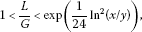(4.4)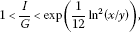(4.5)

where $x,y>0$ with $x\ne y$.

Making use of identity for means

$ln\frac{I}{G}=\frac{A}{L}-1$

given in [34, 35], inequality (4.5) can be changed as follows:

$1<\frac{A}{L}<1+\frac{1}{12}{ln}^{2}\left(x/y\right).$
(4.6)

Employing Theorem 1, Theorem 2 and (2.2), we can prove an interesting chain of inequalities involving the logarithmic mean, identric mean, power mean and geometric mean.

Corollary 3 Let $p\ge 2/3$, $1/\sqrt{5}\le q\le \sqrt{10}/5$, $0. Then the inequalities

$\begin{array}{rcl}G& <& \cdots <{A}_{p}^{1/\left(3p\right)}{G}^{1-1/\left(3p\right)}<\sqrt{IG}<{A}_{q}^{1/\left(3q\right)}{G}^{1-1/\left(3q\right)}
(4.7)

hold for $x,y>0$ with $x\ne y$, where ${I}_{1/2}={I}^{2}\left(\sqrt{x},\sqrt{y}\right)$.

Proof By Remark 1, we see that the function $p↦{A}_{p}^{1/\left(3p\right)}{G}^{1-1/\left(3p\right)}$ is decreasing on $\left(0,\mathrm{\infty }\right)$, and by (2.2) it is deduced that

$G<\cdots <{A}_{p}^{1/\left(3p\right)}{G}^{1-1/\left(3p\right)},\phantom{\rule{2em}{0ex}}{A}_{r}^{1/\left(3r\right)}{G}^{1-1/\left(3r\right)}<\cdots

if $p\ge 2/3$ and $0.

The second and third inequalities are equivalent to (1.17), which hold if and only if $p\ge 2/3$ and $q\le \sqrt{10}/5$ by Theorem 2, respectively.

If $1/\sqrt{5}\le q\le \sqrt{10}/5$, then by Theorem 1 the fourth and fifth inequalities hold.

With $x\to {x}^{1/2}$, $y\to {y}^{1/2}$, $r\to 2r$, by Theorem 2, we have

${A}^{3/2}\left(\sqrt{x},\sqrt{y}\right)
(4.8)

and for $0<2r\le \sqrt{10}/5$, that is, $0,

$\begin{array}{rcl}I\left(\sqrt{x},\sqrt{y}\right)& <& {\left({A}^{1/\left(2r\right)}\left({x}^{1/r},{y}^{1/r}\right)\right)}^{2/\left(3\left(2r\right)\right)}{G}^{1-2/\left(3\left(2r\right)\right)}\left(\sqrt{x},\sqrt{y}\right)\\ =& {\left({A}_{r}^{1/\left(3r\right)}{G}^{1-1/\left(3r\right)}\right)}^{1/2}.\end{array}$
(4.9)

Squaring both sides of (4.8) and (4.9) yields the sixth and seventh inequality, respectively.

The proof is finished. □

From Lemma 6 with (3.4) another known interesting inequality can be reobtained. It should be noted that the second inequality in (4.10) first appeared in  and was reproved by Neuman and Sándor .

Corollary 4 For $x,y>0$ with $x\ne y$, we have

${A}_{2/3}
(4.10)

where $2\sqrt{2}{e}^{-1}$ is the best constant.

Proof From (3.4) it is obtained that

$\underset{t\to \mathrm{\infty }}{lim}g\left(t\right)=\frac{3}{2}ln2-1$

if $p=2/3$. And since the function $t↦g\left(t\right)$ is increasing on $\left(0,\mathrm{\infty }\right)$ by Lemma 6, we have

$0=\underset{t\to \mathrm{\infty }}{lim}g\left(t\right)

that is,

${\left(cosh\frac{2}{3}t\right)}^{3/2}<{e}^{\frac{tcosht}{sinht}-1}<2\sqrt{2}{e}^{-1}{\left(cosh\frac{2}{3}t\right)}^{3/2},$
(4.11)

which is equivalent to (4.10).

Thus the proof is completed. □

## 5 Comparison of some lower bounds for logarithmic mean

As mentioned in the first section of this paper, there are many lower bounds for the logarithmic mean L such as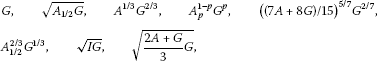and so on, some of which have been proved to be comparable and others remain to be compared further. As applications of our main results, we will discuss them in this section. To this end, we first give a lemma.

Lemma 7 ([, Conclusion 1])

The function $r↦{A}_{r}$ is strictly log-concave on $\left[0,\mathrm{\infty }\right)$.

Now we compare ${A}_{{p}_{0}}^{1/\left(3{p}_{0}\right)}{G}^{1-1/\left(3{p}_{0}\right)}$ with ${\left(\left(7A+8G\right)/15\right)}^{5/7}{G}^{2/7}$.

Lemma 8 Let $p>0$. Then the inequalities

${A}_{{p}_{0}}^{1/\left(3{p}_{0}\right)}{G}^{1-1/\left(3{p}_{0}\right)}>{\left(\frac{7A+8G}{15}\right)}^{5/7}{G}^{2/7}>{A}_{{p}_{1}}^{1/\left(3{p}_{1}\right)}{G}^{1-1/\left(3{p}_{1}\right)}$
(5.1)

hold for all $x,y>0$ with $x\ne y$, where ${p}_{0}=1/\sqrt{5}$ and ${p}_{1}=7/15$ cannot be improved.

Proof By Lemma 1, to prove (5.1), it suffices to show that

$\frac{1}{3{p}_{0}^{2}}ln\left(cosh{p}_{0}t\right)>\frac{5}{7}ln\frac{7cosht+8}{15}>\frac{1}{3{p}_{1}^{2}}ln\left(cosh{p}_{1}t\right).$
(5.2)

For $t>0$, we define

${D}_{1}\left(t\right):=\frac{1}{3{p}^{2}}ln\left(coshpt\right)-\frac{5}{7}ln\frac{7cosht+8}{15}.$

Differentiation and expanding in power series lead to

$\begin{array}{rcl}{D}_{1}^{\mathrm{\prime }}\left(t\right)& =& \frac{\left(8sinhtp+7sinhtpcosht-15pcoshtpsinht\right)}{3p\left(coshtp\right)\left(7cosht+8\right)}\\ =& \frac{\left(7-15p\right)sinht\left(p+1\right)+16sinhtp-\left(7+15p\right)sinht\left(1-p\right)}{6p\left(coshtp\right)\left(7cosht+8\right)}\\ =& \frac{1}{6p\left(coshtp\right)\left(7cosht+8\right)}\sum _{1}^{\mathrm{\infty }}\frac{{p}^{2n-1}{v}_{n}}{\left(2n-1\right)!}{t}^{2n-1},\end{array}$

where

${v}_{n}=\left(7-15p\right){\left(1+\frac{1}{p}\right)}^{2n-1}-\left(7+15p\right){\left(\frac{1}{p}-1\right)}^{2n-1}+16.$

We easily establish a recursive relation for the sequence $\left({v}_{n}\right)$:

${v}_{n+1}={v}_{n}{\left(\frac{1}{p}-1\right)}^{2}+{w}_{n},$
(5.3)

where

${w}_{n}=\frac{4}{p}\left(7-15p\right){\left(1+\frac{1}{p}\right)}^{2n-1}-\frac{16}{p}\left(1-2p\right).$

Clearly, if we prove that for all $n\in \mathbb{N}$, ${v}_{n}\ge 0$ if $p={p}_{0}=1/\sqrt{5}$ and ${v}_{n}\le 0$ if $p={p}_{1}=7/15$, then inequalities (5.1) are valid.

Now we show that for all $n\in \mathbb{N}$, ${v}_{n}\ge 0$ if $p={p}_{0}=1/\sqrt{5}$. In fact, it is easy to verify that ${v}_{1}={v}_{2}=0$, ${v}_{3}=80>0$ and due to $\left(7-15{p}_{0}\right)>0$,

${w}_{n}\ge {w}_{2}=\frac{4}{{p}_{0}}\left(7-15{p}_{0}\right){\left(1+\frac{1}{{p}_{0}}\right)}^{3}-\frac{16}{{p}_{0}^{2}}\left(1-2{p}_{0}\right)=80>0,$

which together with (5.3) yields ${v}_{n+1}>{v}_{n}{\left(\frac{1}{{p}_{0}}-1\right)}^{2}>0$ under the inductive assumption ${v}_{n}>0$ for $n\ge 3$. By mathematical induction, it is acquired that ${v}_{n}\ge 0$ for all $n\in \mathbb{N}$.

We next prove that for all $n\in \mathbb{N}$, ${v}_{n}\le 0$ if $p={p}_{1}=7/15$. It is not difficult to get

${v}_{n}=16-14{\left(\frac{8}{7}\right)}^{2n-1}=16\left(1-{\left(\frac{8}{7}\right)}^{2n-2}\right)\le 0$

for all $n\in \mathbb{N}$.

Lastly, we prove ${p}_{0}=1/\sqrt{5}$ and ${p}_{1}=7/15$ cannot be improved. Indeed, if ${D}_{1}\left(t\right)>0$ for all $t>0$, then we havewhich yields $0. On the other hand, by Lemma 2 the function $p↦\frac{1}{3{p}^{2}}ln\left(coshpt\right)$ is decreasing on $\left(0,\mathrm{\infty }\right)$. Therefore, ${p}_{0}=1/\sqrt{5}$ is the largest constant such that ${D}_{1}\left(t\right)>0$ for all $t>0$.

In the same way, we can prove ${p}_{1}=7/15$ is the smallest constant such that ${D}_{1}\left(t\right)<0$ for all $t>0$.

This completes the proof. □

Next let us compare $\sqrt{\frac{2A+G}{3}G}$ and ${A}_{2/3}^{2/3}{A}_{1/3}^{-1/3}{G}^{2/3}$.

Lemma 9 For $x,y>0$ with $x\ne y$, we have

$\sqrt{\frac{2A+G}{3}G}>{A}_{2/3}^{2/3}{A}_{1/3}^{-1/3}{G}^{2/3}.$
(5.4)

Proof Suppose that $x>y>0$ and let $x/y={t}^{6}$. Then inequality (5.4) can be equivalently changed into

${D}_{2}\left(t\right)=\frac{{t}^{6}+{t}^{3}+1}{3}-t{\left(\frac{{t}^{4}+1}{{t}^{2}+1}\right)}^{2}>0,$

where $t>1$. Simplifying yields

${D}_{2}\left(t\right)=\frac{1}{3{\left({t}^{2}+1\right)}^{2}}{\left(t-1\right)}^{4}\left({t}^{6}+{t}^{5}-{t}^{3}+t+1\right)>0,$

which completes the proof. □

Using Lemmas 7-9, we can easily prove the following.

Proposition 1 For $x,y>0$ with $x\ne y$ and ${p}_{0}=\sqrt{5}/5$, ${p}_{2}=\sqrt{10}/5=0.63246$, we have

$\begin{array}{rcl}L& >& {A}_{{p}_{0}}^{1/\left(3{p}_{0}\right)}{G}^{1-1/\left(3{p}_{0}\right)}>{\left(\frac{7A+8G}{15}\right)}^{5/7}{G}^{2/7}>{A}_{7/15}^{5/7}{G}^{2/7}\\ >& {A}_{1/2}^{2/3}{G}^{1/3}>{A}_{{p}_{2}}^{1/\left(3{p}_{2}\right)}{G}^{1-1/\left(3{p}_{2}\right)}>\sqrt{IG}>\sqrt{{A}_{2/3}G}\\ >& \sqrt{\frac{2A+G}{3}G}>{A}_{2/3}^{2/3}{A}_{1/3}^{-1/3}{G}^{2/3}>{A}^{1/3}{G}^{2/3}.\end{array}$
(5.5)

Proof The first, second and third inequalities follow from Theorem 1 and Lemma 8. Since $7/15<1/2<{p}_{2}=\sqrt{10}/5$, by Theorem 1 the fourth and fifth ones follow. The sixth and seventh ones are obtained from the second and third ones of (4.7).

By the known inequality ${A}_{2/3}>\left(2A+G\right)/3$ (see [, (5.13)]), we easily get the eighth one.

Lemma 9 shows that the ninth one holds.

The last one is equivalent to ${A}_{2/3}>{A}^{1/2}{A}_{1/3}^{1/2}$, which easily follows from Lemma 7.

This completes the proof. □

Lastly, we compare ${A}_{{p}_{0}}^{1/\left(3{p}_{0}\right)}{G}^{1-1/\left(3{p}_{0}\right)}$ with ${A}_{p}^{1-p}{G}^{p}$ ($0).

Proposition 2 Let ${p}_{0}=1/\sqrt{5}$ and $0. Then

${A}_{{p}_{0}}^{1/\left(3{p}_{0}\right)}{G}^{1-1/\left(3{p}_{0}\right)}>{A}_{p}^{1-p}{G}^{p}$
(5.6)

holds for all $x,y>0$ with $x\ne y$ if $1-1/\left(3{p}_{0}\right)\le p<1$. While ${A}_{{p}_{0}}^{1/\left(3{p}_{0}\right)}{G}^{1-1/\left(3{p}_{0}\right)}$ and ${A}_{p}^{1-p}{G}^{p}$ are not comparable if $0.

Proof (i) By a simple equivalent transformation, inequality (5.6) can be changed into

${A}_{{p}_{0}}>{A}_{p}^{3{p}_{0}\left(1-p\right)}{G}^{3{p}_{0}p-3{p}_{0}+1}:={A}_{p}^{\alpha }{A}_{0}^{\beta },$

where $\alpha =3{p}_{0}\left(1-p\right)$, $\beta =3{p}_{0}p-3{p}_{0}+1$. If $1-1/\left(3{p}_{0}\right)\le p<1$, then $\alpha \ge 0$, $\beta >0$ with $\alpha +\beta =1$. By Lemma 7, it is derived that

${A}_{p}^{\alpha }{A}_{0}^{\beta }<{A}_{\alpha p}={A}_{3{p}_{0}\left(1-p\right)p},$

and using the basic inequality $\left(1-p\right)p\le 1/4$ for $0 and the monotonicity of the function $r↦{A}_{r}$, we get

${A}_{3{p}_{0}\left(1-p\right)p}\le {A}_{3{p}_{0}/4}<{A}_{{p}_{0}},$

which proves inequality (5.6).

(ii) We define

${D}_{3}\left(t\right):=\frac{1}{3{p}_{0}^{2}}ln\left(cosh{p}_{0}t\right)-\frac{1-p}{p}lncoshpt.$

By Lemma 1, to show that ${A}_{{p}_{0}}^{1/\left(3{p}_{0}\right)}{G}^{1-1/\left(3{p}_{0}\right)}$ and ${A}_{p}^{1-p}{G}^{p}$ are not comparable if $0, we need to illustrate $sgn\left({D}_{3}\left(t\right)\right)$ is not a constant. In fact, utilizing L’Hospital’s rule, we have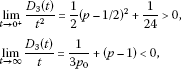which implies that there are numbers ${t}_{2}>{t}_{1}>0$ such that ${D}_{3}\left(t\right)>0$ when $t\in \left(0,{t}_{1}\right)$ and ${D}_{3}\left(t\right)<0$ when $t\in \left({t}_{2},\mathrm{\infty }\right)$. Consequently, $\frac{1}{3{p}_{0}^{2}}ln\left(cosh{p}_{0}t\right)$ and $\frac{1-p}{p}lncoshpt$ are not comparable on $\left(0,\mathrm{\infty }\right)$ if $0, which is the desired result.

Thus the proof is finished. □

Remark 3 From the above two propositions, as far as the lower bounds for the logarithmic mean are concerned, our new lower bound ${A}_{{p}_{0}}^{1/\left(3{p}_{0}\right)}{G}^{1-1/\left(3{p}_{0}\right)}$ seems to be superior to most known ones.

## References

1. Bullen PS, Mitrinović DS, Vasić PM: Means and Their Inequalities. Reidel, Dordrecht; 1988.

2. Ostle B, Terwilliger HL: A comparison of two means. Proc. Mont. Acad. Sci. 1957, 17: 69–70.

3. Karamata J: Sur quélques problémes posés par Ramanujan. J. Indian Math. Soc. 1960, 24: 343–365.

4. Mitrinović DS: Analytic Inequalities. Springer, New York; 1970.

5. Yang Z-H: Other property of the convex function. Chin. Math. Bull. 1984, 2: 31–32. (in Chinese)

6. Yang Z-H: Exponential mean and logarithmic mean. Math. Pract. Theory 1987, 4: 76–78. (in Chinese)

7. Sándor J: Some integral inequalities. Elem. Math. 1988, 43: 177–180.

8. Lin TP: The power mean and the logarithmic mean. Am. Math. Mon. 1974, 81: 879–883. 10.2307/2319447

9. Neuman E: The weighted logarithmic mean. J. Math. Anal. Appl. 1994, 188: 885–900. 10.1006/jmaa.1994.1469

10. Carlson BC: The logarithmic mean. Am. Math. Mon. 1972, 79: 615–618. 10.2307/2317088

11. Leach EB, Sholander MC: Extended mean values II. J. Math. Anal. Appl. 1983, 92: 207–223. 10.1016/0022-247X(83)90280-9

12. Wang C, Wang X: Quadrature formulae and analytic inequalities - on the separation of power means by logarithmic mean. Hangzhou Daxue Xuebao 1982, 9(2):156–159.

13. Chen J, Wang Z: On the lower bound of the logarithmic mean. Chengdu Ke-Ji Daxue Xuebao 1990, 1990(2):100–102.

14. Zhu L: Generalized Lazarevićs inequality and its applications - Part II. J. Inequal. Appl. 2009., 2009: Article ID 379142

15. Alzer H: Two inequalities for means. C. R. Math. Acad. Sci. 1987, 9: 11–16.

16. Yang Z-H: Some monotonicity results for certain mixed means with parameters. Int. J. Appl. Math. Sci. 2012., 2012: Article ID 540710. doi:10.1155/2012/540710

17. Stolarsky KB: Generalizations of the logarithmic mean. Math. Mag. 1975, 48: 87–92. 10.2307/2689825

18. Stolarsky KB: The power and generalized logarithmic means. Am. Math. Mon. 1980, 87: 545–548. 10.2307/2321420

19. Pittenger AO: The symmetric, logarithmic and power means. Publ. Elektroteh. Fak. Univ. Beogr., Ser. Mat. Fiz. 1980, 1980(678–715):19–23.

20. Sándor J: A note on some inequalities for means. Arch. Math. 1991, 56: 471–473. 10.1007/BF01200091

21. Allasia G, Giordano C, Pěcarić J: On the arithmetic and logarithmic means with applications to Stirling’s formula. Atti Semin. Mat. Fis. Univ. Modena 1999, 47: 441–455.

22. Alzer H, Qiu S-L: Inequalities for means in two variables. Arch. Math. 2003, 80: 201–215. 10.1007/s00013-003-0456-2

23. Burk F: The geometric, logarithmic, and arithmetic mean inequality. Am. Math. Mon. 1987, 94: 527–528. 10.2307/2322844

24. Kouba O: New bounds for the identric mean of two arguments. J. Inequal. Pure Appl. Math. 2008., 9(3): Article ID 71

25. Neuman E, Sándor J: On the Schwab-Borchardt mean. Math. Pannon. 2003, 14: 253–266.

26. Neuman E, Sándor J: On certain means of two arguments and their extensions. Int. J. Math. Math. Sci. 2003, 2003(16):981–993. 10.1155/S0161171203208103

27. Sándor J: Inequalities for means. Proc. 3rd Symposium of Math. and Its Appl. 1989, 87–90.

28. Sándor J: On refinements of certain inequalities for means. Arch. Math. 1995, 31: 279–282.

29. Sándor J, Rasa I: Inequalities for certain means in two arguments. Nieuw Arch. Wiskd. 1997, 15: 51–55.

30. Sándor J, Trif T: Some new inequalities for means of two arguments. Int. J. Math. Math. Sci. 2001, 25: 525–532. 10.1155/S0161171201003064

31. Vamanamurthy MK, Vuorinen M: Inequalities for means. J. Math. Anal. Appl. 1994, 183: 155–166. 10.1006/jmaa.1994.1137

32. Xia W-F, Chu Y-M, Wang G-D: The optimal upper and lower power mean bounds for a convex combination of the arithmetic and logarithmic means. Abstr. Appl. Anal. 2010., 2010: Article ID 604804

33. Yang Z-H: ON the log-convexity of two-parameter homogeneous functions. Math. Inequal. Appl. 2007, 10(3):499–516.

34. Seiffert H-J: Comment to problem 1365. Math. Mag. 1992, 65: 356. 10.2307/2691251

35. Yang Z-H: Some identities for means and applications. RGMIA Research Report Collection 2005., 8(3): Article ID 17. Available online at http://www.ajmaa.org/RGMIA/papers/v8n3/imp.pdf

36. Neuman E, Sándor J: Companion inequalities for certain bivariate means. Appl. Anal. Discrete Math. 2009, 3(1):46–51. Available online at http://www.doiserbia.nb.rs/img/doi/1452–8630/2009/1452–86300901046N.pdf 10.2298/AADM0901046N

## Acknowledgements

The author would like to thank Mr. Pi for her help. The author also wishes to thank the reviewer(s) who gave some important and valuable advises.

## Author information

Authors

### Corresponding author

Correspondence to Zhen-Hang Yang.

### Competing interests

The author declares that they have no competing interests.

## Rights and permissions

Reprints and Permissions

Yang, ZH. New sharp bounds for logarithmic mean and identric mean. J Inequal Appl 2013, 116 (2013). https://doi.org/10.1186/1029-242X-2013-116

• Accepted:

• Published:

• DOI: https://doi.org/10.1186/1029-242X-2013-116

### Keywords

• logarithmic mean
• identric mean
• power mean
• inequality